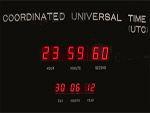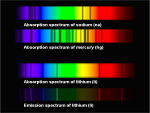Automatic translation Category: matter and particles
Updated Nov 16, 2015### Image: The Flagellation of Christ by Piero Della Francesca (between 1444 and 1478). The work of Piero Della Francesca, master of perspective and Euclidean geometry, also reproduced perfectly as possible the space taken up by the human eye. From the beginning of the renaissance space becomes more abstract, can be seen from the outside. The synthetic Euclidean geometry then presents a problem, in particular, the axiom of parallels (Euclid's fifth postulate).### Image: It is easy to visualize the Möbius strip in space. It is a simple model to achieve, just do a twist to a strip of paper and then pasting its two ends. And there can be seen that the tape has only one side, unlike a conventional tape which has two. Mobius strip provided of the projection on a mediator circle is a fiber space. This is a simple example of abstract manifold but beyond two dimensions this is no longer representable. Thus the Klein bottle, a closed surface without boundary and not adjustable in 3-dimensional space, has no "inside" or "outside". credit: GNU Free Documentation License

The wavelength of de Broglie is a concept of quantum mechanics.
This is a quantity that represents the thermal wavelength characterizing the spatial spread of a particle.

λ = h/(mv)
h is Planck's constant
m is the mass of the particle
v is the speed

When a gas is cooled, it decreases the speed of the atoms, the wave nature comes in.
When the speed decreases the wavelength of de Broglie increases thus the wave packet associated with each particle, become increasingly "large", the wavelength is spread out more and more from growing. In other words, low temperature = low speed so high wavelength de Broglie thus high spatial spreading of the particle.
Space-time is a mathematical manifold to 4 dimensions, three spatial dimensions and one time dimension, time is geometrized, it flows on a straight line, it is the fourth dimension of space.
In addition, space-time has a property that is the curvature and it varies from one place to another and each point in space has a radius of curvature.
This curvature is gravitation.
Thus, all space objects feel the curvature of space-time.
A tensor is a very complex abstract mathematical object that has an arbitrary number of primed indices and unprimed indices.
Compared to the tensor, matrix is a simple array of numbers, while the tensor is as vectors, an abstract object whose coordinates change when going from a representation in a given base to a representation in another base.
For example, in differential geometry, the tensor allows to express the curvature. In a curved space, parallel are not necessarily preserved. More space is curved more geodesic will be towards or away quickly.
An axiom means an unprovable truth that must be accepted.
The axiom is used as a basis for reasoning.
Some Greek philosophers of antiquity, an axiom was a statement they considered obvious and needed no proof.

1997 © Astronoo.com − Astronomy, Astrophysics, Evolution and Earth science.Windowsof the spectrum...Leap second...Cosmologicalconstants...